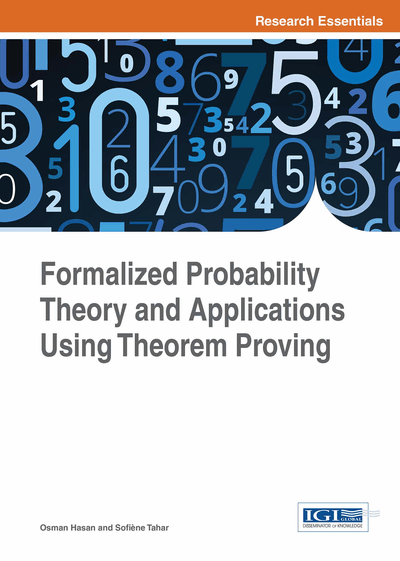# Formalization of Hidden Markov Model

DOI: 10.4018/978-1-4666-8315-0.ch008
Available
\$37.50
No Current Special Offers

## Abstract

In this chapter, the authors provide the formalization of extended DTMC models, namely Hidden Markov Models (HMMs), which are the core concept for formally evaluating the probability of the occurrence of a particular observed sequence and finding the best state sequence to generate given observation (Mantyla & Tutkimuskeskus, 2001; Rabiner, 1990). In order to present the usefulness of the formalization of HMM and the formal verification of HMM properties, the authors illustrate the formal analysis of a DNA (Deoxyribon Nucleic Acid) sequence at the end of the chapter.
Chapter Preview
Top

## 8.1 Definition Of Hmm

In order to accurately analyze the HMMs (Eddy, 2004), we propose to apply the formalized DTMC to formally define HMMs and verify their properties in higher-order logic as the extended DTMC models.

An HMM is a pair of two stochastic processes { Xk; Yk } k ≥ 0, where { Xk } k ≥ 0 is a Markov chain, and { Yk } k ≥ 0 is conditionally independent of { Xk }, i.e., Yk depends only on Xk and not on any Xt, such that t ≠ k. The HMMs model situations where an experimenter sees some observers at every instant (mathematically represented by Yk) and suspects these observables to be the outcome of a process that can be modeled by a Markov chain ({ Xk } k ≥ 0). The name “Hidden Markov Model” arises from the fact that the state in which this model is at a particular instant is not available to the observer. Now, a HMM is defined as a parameterized triple (A, B, π (0)) with the following conditions:

• 1.

Hidden Markov Chain { Xk } k ≥ 0 with a finite state space S, the initial distribution π (0) = { π i (0) } i є S and the transition probabilities A = { aij } i є S, j є S.

• 2.

A random process { Yk } k ≥ 0 with finite state space O. The hidden Markov chain and the random process are associated with the emission probabilities B = { bj (Ok) } j є S,k є O = { Pr { Yn = Ok | Xn = j } } j є S, k є O. It implies that:

• a.

∀ j k. bj (Ok) ≥0,

• b.

∑ k є Obj (Ok) = 1.

• 3.

The random process { Yk } k ≥ 0 and hidden Markov chain { Xk } k ≥ 0 have conditional independence.

This yields the formalization (Liu, 2013):

• Definition 8.1

```⊢ ∀ X Y p sX sY p0 pij pXY.
hmm X Y p sX sY p0 pij pXY =
dtmc X p sX p0 pij∧ (∀t. random variable (Y t) p sY) ∧
(∀ i. i ∈ space sY{ i } ∈ subsets sY) ∧
(∀ t a i. ℙ { x | X t x = I } ≠ 0 ⇒
ℙ ({ x | Y t x = a } | { x | X t x = I }) = pXY t a I) ∧
∀ t a i tx0 ty0 stsX stsY tsX tsY.
t ∉ { tx0 + m | m ∈ tsX } ∧ t ∉ { ty0 + m | m ∈ tsY } ∧
ℙ ({ x | X t x = I } ∩k є t sX { x | X (tx0 + k) x = EL k stsX } ∩
k є t sY{ x | Y (ty0 + k) x = EL k stsY }) ≠ 0 ⇒
ℙ ({ x | Y t x = a } |
({ x | X t x = I } ∩ k є t sX { x | X (tx0 + k) x = EL k stsX } ∩
k є t sY { x | Y (ty0 + k) x = EL k stsY })) =
ℙ ({ x | Y t x = a } | { x | X t x = I })```

In this definition, the variable X denotes the random variable of the underlying DTMC (as the first conjunct constrains), Y indicates the random observations (so Y t is a random process as the second condition describes), and pXY indicates the emission probabilities, i.e., the probability of obtaining a particular value for Y depending on the state X. Like the second condition in Definition 6.3, the condition (∀ i. i ∈ space sY ⇒ { I } ∈ subsets sY) ensures that the event space is a discrete space. The conjunct (∀ t a i. ℙ { x | X t x = I } ≠ 0 ⇒ ℙ ({ x | Y t x = a } | { x | X t x = I }) = pXY t a I) assigns the function pXY to emission probabilities under the condition ℙ { x | X t x = I } ≠ 0, which ensures that the corresponding conditional probabilities are well-defined. The non-trivial conjunct in the above definition is the last one which formalizes the notion of conditional independence mentioned above. In our work, we consider mainly discrete time and finite-state space HMMs, which is the most frequently used case.

Time-homogenous HMMs can also be formalized in a way similar to time homogenous DTMCs (Liu, 2013). Note that, in practice, time-homogenous HMMs always have a finite state-space (Liu, Aravantinos, Hasan & Tahar, 2014).

• Definition 8.2

## Complete Chapter List

Search this Book:
Reset